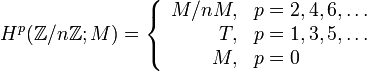# Group cohomology of finite cyclic groups

Jump to: navigation, search
This article gives specific information, namely, group cohomology, about a family of groups, namely: finite cyclic group.
View group cohomology of group families | View other specific information about finite cyclic group

## Classifying space and corresponding chain complex

The classifying space of a finite cyclic group of order$n$ is a lens space (Read more about the lens space as a topological space on the Topology Wiki).

A chain complex that can be used to compute the homology and cohomology for the classifying space and hence also for the group is:$\dots \stackrel{\cdot 0}{\to} \mathbb{Z} \stackrel{\cdot n}{\to} \mathbb{Z} \stackrel{\cdot 0}{\to} \mathbb{Z} \to \dots \stackrel{\cdot n}{\to} \mathbb{Z} \stackrel{\cdot 0}{\to} \mathbb{Z}$

where the subscript for the last written entry is$0$, and hence the multiplication by$n$ maps arise from even to odd subscripts and the multiplication by zero maps arise from odd to even subscripts.

## Homology groups

To look at the same material from a topological/algebraic topology perspective, check out the homology of lens space at the Topology Wiki

### Over the integers

The homology groups with coefficients in the ring of integers$\mathbb{Z}$ are given as follows:$H_p(\mathbb{Z}/n\mathbb{Z};\mathbb{Z}) = \left\lbrace\begin{array}{rl}\mathbb{Z}/n\mathbb{Z}, &p = 1,3,5,\dots\\0, & p = 2,4,6, \dots \\ \mathbb{Z},& p = 0\\\end{array}\right.$

### Over an abelian group

The homology groups with coefficients in an abelian group$M$ (which we may treat as a module over a unital ring$R$, which could be$\mathbb{Z}$ or something else) are given by:$H_p(\mathbb{Z}/n\mathbb{Z};M) = \left\lbrace\begin{array}{rl} M/nM, & p=1,3,5,\dots\\ T, & p = 2,4,6, \dots \\ M, & p = 0\\\end{array}\right.$

where$T$ is the$n$-torsion submodule of$M$, i.e., the submodule of$M$ comprising elements which, when multiplied by$n$, give zero.

In particular, we see the following cases:

Case on$R$ or$M$ Conclusion about odd-indexed homology groups, i.e.,$H_p, p = 1,3,5,\dots$ Conclusion about even-indexed homology groups, i.e.,$H_p, p = 2,4,6,\dots$$M$ is uniquely$n$-divisible, i.e., every element of$M$ can be uniquely divided by$n$. This includes the case that$M$ is a field of characteristic not dividing$n$. all zero groups all zero groups$M$ is$n$-torsion-free, i.e., no nonzero element of$M$ doubles to zero unclear all zero groups$M$ is$n$-divisible, but not necessarily uniquely so, e.g.,$M = \mathbb{Q}/\mathbb{Z}$ all zero groups unclear

## Cohomology groups

The cohomology groups with coefficients in the ring of integers$\mathbb{Z}$ are given as follows:$H^p(\mathbb{Z}/n\mathbb{Z};\mathbb{Z}) = \left\lbrace\begin{array}{rl}\mathbb{Z}/n\mathbb{Z}, &p = 2,4,6,\dots\\0, & p = 1,3,5, \dots \\ \mathbb{Z},& p = 0\\\end{array}\right.$

### Over an abelian group

The cohomology groups with coefficients in an abelian group$M$ (which we may treat as a module over a unital ring$R$, which could be$\mathbb{Z}$ or something else) are given by:$H^p(\mathbb{Z}/n\mathbb{Z};M) = \left\lbrace\begin{array}{rl} M/nM, & p=2,4,6,\dots\\ T, & p = 1,3,5, \dots \\ M, & p = 0\\\end{array}\right.$

where$T$ is the$n$-torsion submodule of$M$, i.e., the submodule of$M$ comprising elements which, when multiplied by$n$, give zero.